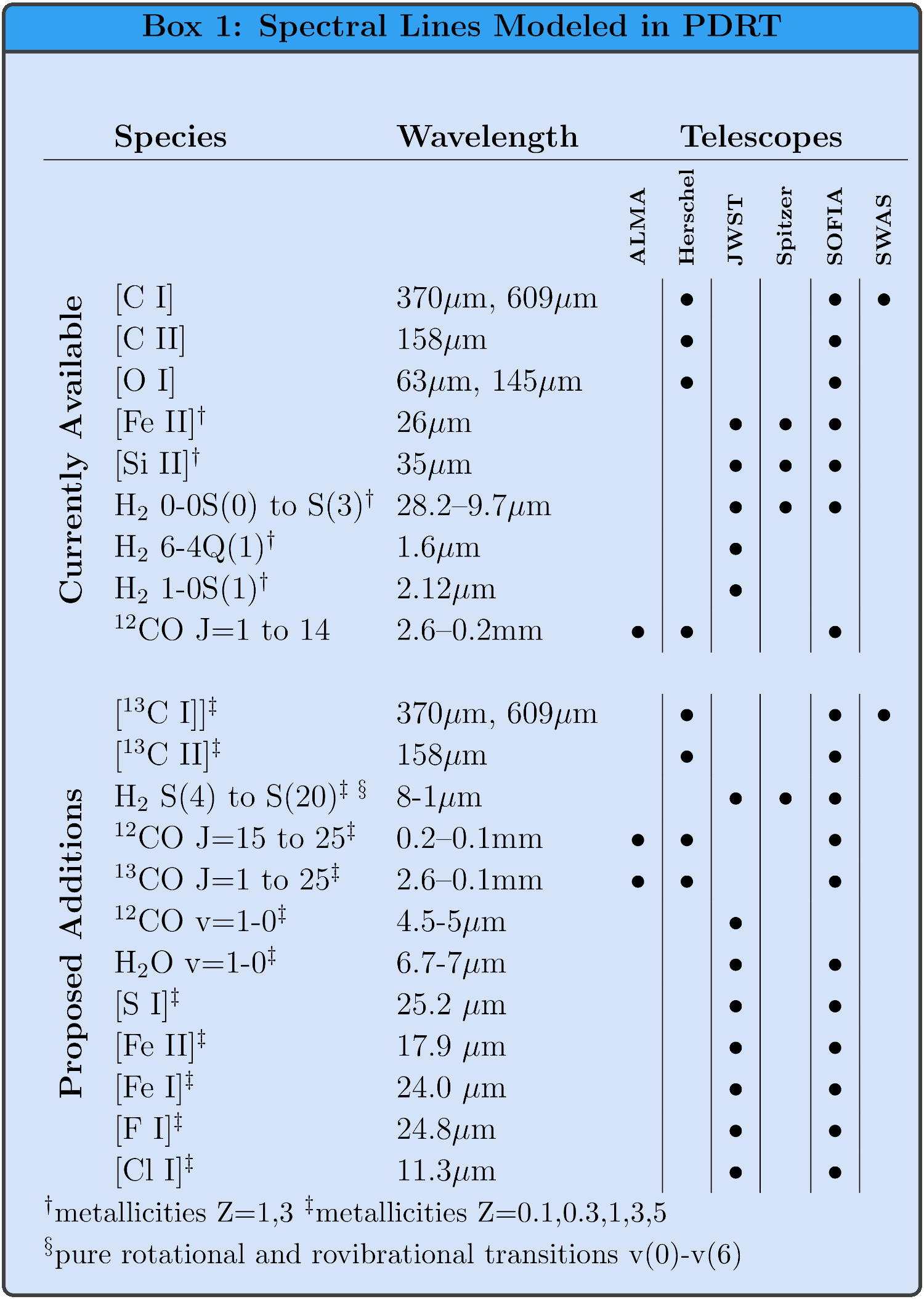# The Models

The models are based on the work of Tielens & Hollenbach 1985; Wolfire et al. 1990; Hollenbach et al. 1991; Kaufman et al. 1999; and Kaufman et al. 2006. For a given set of gas phase elemental abundances and grain properties, each model is described by a constant H nucleus number density, $$n$$, or constant thermal pressure, $$P_{th}$$, and incident far-ultraviolet intensity $$G_0$$. The models solve for the chemical balance, thermal equilibrium, and radiation transfer through a PDR layer. Our treatment updates previous results to use recent values of atomic and molecular data, current chemical rate coefficients, and grain photolectric heating rates. The are a number model data sets ("ModelSets") currently available in PDR Toolbox. The ModelSets come in two basic flavors: constant density and constant thermal pressure. The constant density ModelSets present spectral line and continuum intensity ratios as a function of $$G_0$$ and $$n$$. The constant thermal pressure ModelSets present the intensity ratios as a function of $$G_0$$ and thermal pressure $$P_{th}$$. PDR Toolbox will also support importing the external models of your choice, provided they are stored in the right format.

The ratio plots are used to determine the beam averaged values of $$G_0$$ and $$n$$. Note that $$n$$ is the H nucleus density (cm-3). so that the maximum abundance of H2, for example, is $$n(H_2)= 0.5~n$$. The values of $$G_0$$ and $$n$$ are obtained by fitting the observations to the model space. Required are at least two observations of line intensity ratios or one line intensity ratio and the ratio of the [C II] + [O I] intensity to the infrared dust continuum emission $$I_{FIR}$$. More than two observed ratios will of course do a better job of determining unique $$G_0$$ and $$n$$.

It is important to correct for the different beam area filling factors for various species. For example, diffuse [C II] emission may fill the observing beam whereas [O I] emission may arise from a smaller, high density and high temperature region. In such a case, it would not be appropriate to take the ratio of observed fluxes for fitting. Instead the ratio of intensities should be used or the ratio of fluxes corrected for the separate emitting solid angles. Note also that the ([C II] + [O I])/$$I_{FIR}$$ model ratios are for a single face-on PDR. For the case of active regions of galaxies, or where there may be many clouds contained within the beam, the FIR continuum is observed from both the near and far side of the cloud. Thus, the observed ratio should be multiplied by a factor 2 (or equivalently the model values should be divided by 2).

## Model Specifics

#### 2006 Models

These models are identical to those available in the "classic" web-based PDR Toolbox. They have metallicity $$Z=1$$, and contain [C I], [C II], [O I], Fe, Si, H2 lines and the 12CO ladder up to $$J=14\rightarrow 13$$. For some lines, $$Z=3$$ is also available.

In pdrtpy, these are referred to as the wk2006 ModelSet.

#### 2020 Models

These model data start with the 2006 PDR model code and add improved physics and chemistry. Critical updates include those discussed in Neufeld & Wolfire 2016, plus photo rates from Heays et al. 2017, oxygen chemistry rates from Kovalenko et al. 2018 and Tran et al. 2018, and carbon chemistry rates from Dagdigian 2019. We have also implemented new collisional excitation rates for [O I] from Lique et al. 2018 (and Lique private communication) and have included 13C chemistry along with the emitted line intensities for [13C II] and 13CO. In Box 1, we show the currently available spectral lines and those we expect to deploy later in the project. In addition, we will compute ModelSets for a full range of metallicity $$Z$$.

In pdrtpy, these are referred to as the wk2020 ModelSet. As of August 2020, we are still computing these models and make subsets available in pdrtpy as they become ready for use.Current and future spectral line and metallicity coverage of the PDR Toolbox.

#### Model Parameters

Our 2006 models use the standard parameters from Kaufman et al. 1999. Changes for the 2020 models are listed below. The formation rate of H2 goes as $$R_{form}~n_{HI}~n$$. The PAH abundance $$X_{PAH}=n_{PAH}/n$$.

###### Parameters for 2006 Models
Parameter Symbol Value
Turbulent Doppler velocity $$\delta v_D$$ 1.5 km s-1
Carbon abundance $$X_C$$ $$1.4\times10^{-4}$$
Oxygen abundance $$X_O$$ $$3.0\times10^{-4}$$
Silicon abundance $$X_{Si}$$ $$1.7\times10^{-6}$$
Sulfur abundance $$X_{S}$$ $$2.8\times10^{-5}$$
Iron abundance $$X_{Fe}$$ $$1.7\times10^{-7}$$
Magnesium abundance $$X_{Mg}$$ $$1.1\times10^{-6}$$
Dust abundance relative to diffuse ISM $$\delta_d$$ 1
FUV dust absorption/visual extinction $$\delta_{UV}$$ 1.8
Dust visual extinction per H $$\sigma_V$$ $$5\times10^{-22}$$ cm-2
Formation rate of H2 on dust $$R_{form}$$ $$3\times10^{-17}$$ s-1
PAH abundance $$X_{PAH}$$ $$5\times10^{-7}$$
Cloud H density $$n$$ $$10^{1} - 10^{7}$$ cm-3
Incident UV flux $$G_0$$ $$10^{-0.5} - 10^{6.5}$$ Habing
###### Parameters for 2020 Models Changed from 2006
Parameter Symbol Value
Carbon abundance $$X_C$$ $$1.6\times10^{-4}$$
Oxygen abundance $$X_O$$ $$3.2\times10^{-4}$$
Dust visual extinction per H $$\sigma_V$$ $$5.26\times10^{-22}$$ cm-2
Formation rate of H2 on dust $$R_{form}$$ $$6\times10^{-17}$$ s-1
PAH abundance $$X_{PAH}$$ $$2\times10^{-7}$$